# 8.2 Multiply and divide rational expressions

 Page 3 / 3

Divide: $\frac{\frac{6{x}^{2}-7x+2}{4x-8}}{\frac{2{x}^{2}-7x+3}{{x}^{2}-5x+6}}.$

## Solution

$\begin{array}{cccc}& & & \hfill \phantom{\rule{5em}{0ex}}\frac{\frac{6{x}^{2}-7x+2}{4x-8}}{\frac{2{x}^{2}-7x+3}{{x}^{2}-5x+6}}\hfill \\ \\ \\ \text{Rewrite with a division sign.}\hfill & & & \phantom{\rule{5em}{0ex}}\frac{6{x}^{2}-7x+2}{4x-8}÷\frac{2{x}^{2}-7x+3}{{x}^{2}-5x+6}\hfill \\ \\ \\ \begin{array}{c}\text{Rewrite as product of first times}\hfill \\ \text{reciprocal of second.}\hfill \end{array}\hfill & & & \phantom{\rule{5em}{0ex}}\frac{6{x}^{2}-7x+2}{4x-8}·\frac{{x}^{2}-5x+6}{2{x}^{2}-7x+3}\hfill \\ \\ \\ \begin{array}{c}\text{Factor the numerators and the}\hfill \\ \text{denominators, and then multiply.}\hfill \end{array}\hfill & & & \phantom{\rule{5em}{0ex}}\frac{\left(2x-1\right)\left(3x-2\right)\left(x-2\right)\left(x-3\right)}{4\left(x-2\right)\left(2x-1\right)\left(x-3\right)}\hfill \\ \\ \\ \text{Simplify by dividing out common factors.}\hfill & & & \phantom{\rule{5em}{0ex}}\frac{\overline{)\left(2x-1\right)}\left(3x-2\right)\overline{)\left(x-2\right)}\overline{)\left(x-3\right)}}{4\overline{)\left(x-2\right)}\overline{)\left(2x-1\right)}\overline{)\left(x-3\right)}}\hfill \\ \\ \\ \text{Simplify.}\hfill & & & \hfill \phantom{\rule{5em}{0ex}}\frac{3x-2}{4}\hfill \end{array}$

Divide: $\frac{\frac{3{x}^{2}+7x+2}{4x+24}}{\frac{3{x}^{2}-14x-5}{{x}^{2}+x-30}}.$

$\frac{x+2}{4}$

Divide: $\frac{\frac{{y}^{2}-36}{2{y}^{2}+11y-6}}{\frac{2{y}^{2}-2y-60}{8y-4}}.$

$\frac{2}{y+5}$

If we have more than two rational expressions to work with, we still follow the same procedure. The first step will be to rewrite any division as multiplication by the reciprocal. Then we factor and multiply.

Divide: $\frac{3x-6}{4x-4}·\frac{{x}^{2}+2x-3}{{x}^{2}-3x-10}÷\frac{2x+12}{8x+16}.$

## Solution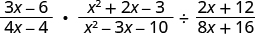Rewrite the division as multiplication by the reciprocal.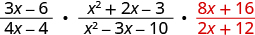Factor the numerators and the denominators, and then multiply.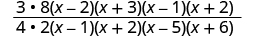Simplify by dividing out common factors.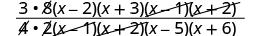Simplify.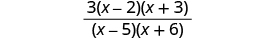Divide: $\frac{4m+4}{3m-15}·\frac{{m}^{2}-3m-10}{{m}^{2}-4m-32}÷\frac{12m-36}{6m-48}.$

$\frac{2\left(m+1\right)\left(m+2\right)}{3\left(m+4\right)\left(m-3\right)}$

Divide: $\frac{2{n}^{2}+10n}{n-1}÷\frac{{n}^{2}+10n+24}{{n}^{2}+8n-9}·\frac{n+4}{8{n}^{2}+12n}.$

$\frac{\left(n+5\right)\left(n+9\right)}{2\left(n+6\right)\left(2n+3\right)}$

## Key concepts

• Multiplication of Rational Expressions
• If $p,q,r,s$ are polynomials where $q\ne 0,s\ne 0$ , then $\frac{p}{q}·\frac{r}{s}=\frac{pr}{qs}$ .
• To multiply rational expressions, multiply the numerators and multiply the denominators
• Multiply a Rational Expression
1. Factor each numerator and denominator completely.
2. Multiply the numerators and denominators.
3. Simplify by dividing out common factors.
• Division of Rational Expressions
• If $p,q,r,s$ are polynomials where $q\ne 0,r\ne 0,s\ne 0$ , then $\frac{p}{q}÷\frac{r}{s}=\frac{p}{q}·\frac{s}{r}$ .
• To divide rational expressions multiply the first fraction by the reciprocal of the second.
• Divide Rational Expressions
1. Rewrite the division as the product of the first rational expression and the reciprocal of the second.
2. Factor the numerators and denominators completely.
3. Multiply the numerators and denominators together.

4. Simplify by dividing out common factors.

## Practice makes perfect

Multiply Rational Expressions

In the following exercises, multiply.

$\frac{12}{16}·\frac{4}{10}$

$\frac{3}{10}$

$\frac{32}{5}·\frac{16}{24}$

$\frac{18}{10}·\frac{4}{30}$

$\frac{6}{25}$

$\frac{21}{36}·\frac{45}{24}$

$\frac{5{x}^{2}{y}^{4}}{12x{y}^{3}}·\frac{6{x}^{2}}{20{y}^{2}}$

$\frac{{x}^{3}}{8y}$

$\frac{8{w}^{3}y}{9{y}^{2}}·\frac{3y}{4{w}^{4}}$

$\frac{12{a}^{3}b}{{b}^{2}}·\frac{2a{b}^{2}}{9{b}^{3}}$

$\frac{8ab}{3}$

$\frac{4m{n}^{2}}{5{n}^{3}}·\frac{m{n}^{3}}{8{m}^{2}{n}^{2}}$

$\frac{5{p}^{2}}{{p}^{2}-5p-36}·\frac{{p}^{2}-16}{10p}$

$\frac{p\left(p-4\right)}{2\left(p-9\right)}$

$\frac{3{q}^{2}}{{q}^{2}+q-6}·\frac{{q}^{2}-9}{9q}$

$\frac{4r}{{r}^{2}-3r-10}·\frac{{r}^{2}-25}{8{r}^{2}}$

$\frac{r+5}{2r\left(r+2\right)}$

$\frac{s}{{s}^{2}-9s+14}·\frac{{s}^{2}-49}{7{s}^{2}}$

$\frac{{x}^{2}-7x}{{x}^{2}+6x+9}·\frac{x+3}{4x}$

$\frac{x-7}{4\left(x+3\right)}$

$\frac{2{y}^{2}-10y}{{y}^{2}+10y+25}·\frac{y+5}{6y}$

$\frac{{z}^{2}+3z}{{z}^{2}-3z-4}·\frac{z-4}{{z}^{2}}$

$\frac{z+3}{z\left(z+1\right)}$

$\frac{2{a}^{2}+8a}{{a}^{2}-9a+20}·\frac{a-5}{{a}^{2}}$

$\frac{28-4b}{3b-3}·\frac{{b}^{2}+8b-9}{{b}^{2}-49}$

$-\frac{4\left(b+9\right)}{3\left(b+7\right)}$

$\frac{18c-2{c}^{2}}{6c+30}·\frac{{c}^{2}+7c+10}{{c}^{2}-81}$

$\frac{35d-7{d}^{2}}{{d}^{2}+7d}·\frac{{d}^{2}+12d+35}{{d}^{2}-25}$

$-7$

$\frac{72m-12{m}^{2}}{8m+32}·\frac{{m}^{2}+10m+24}{{m}^{2}-36}$

$\frac{4n+20}{{n}^{2}+n-20}·\frac{{n}^{2}-16}{4n+16}$

$1$

$\frac{6{p}^{2}-6p}{{p}^{2}+7p-18}·\frac{{p}^{2}-81}{3{p}^{2}-27p}$

$\frac{{q}^{2}-2q}{{q}^{2}+6q-16}·\frac{{q}^{2}-64}{{q}^{2}-8q}$

$1$

$\frac{2{r}^{2}-2r}{{r}^{2}+4r-5}·\frac{{r}^{2}-25}{2{r}^{2}-10r}$

Divide Rational Expressions

In the following exercises, divide.

$\frac{t-6}{3-t}÷\frac{{t}^{2}-9}{t-5}$

$-\frac{2t}{{t}^{3}-5t-9}$

$\frac{v-5}{11-v}÷\frac{{v}^{2}-25}{v-11}$

$\frac{10+w}{w-8}÷\frac{100-{w}^{2}}{8-w}$

$-\frac{1}{10-w}$

$\frac{7+x}{x-6}÷{\frac{49-x}{x+6}}^{2}$

$\frac{27{y}^{2}}{3y-21}÷\frac{3{y}^{2}+18}{{y}^{2}+13y+42}$

$\frac{3{y}^{2}\left(y+6\right)\left(y+7\right)}{\left(y-7\right)\left({y}^{2}+6\right)}$

$\frac{24{z}^{2}}{2z-8}÷\frac{4z-28}{{z}^{2}-11z+28}$

$\frac{16{a}^{2}}{4a+36}÷\frac{4{a}^{2}-24a}{{a}^{2}+4a-45}$

$\frac{a\left(a-5\right)}{a-6}$

$\frac{24{b}^{2}}{2b-4}÷\frac{12{b}^{2}+36b}{{b}^{2}-11b+18}$

$\frac{5{c}^{2}+9c+2}{{c}^{2}-25}÷\frac{3{c}^{2}-14c-5}{{c}^{2}+10c+25}$

$\frac{\left(c+2\right)\left(c+2\right)}{\left(c-2\right)\left(c-3\right)}$

$\frac{2{d}^{2}+d-3}{{d}^{2}-16}÷\frac{2{d}^{2}-9d-18}{{d}^{2}-8d+16}$

$\frac{6{m}^{2}-2m-10}{9-{m}^{2}}÷\frac{6{m}^{2}+29m-20}{{m}^{2}-6m+9}$

$-\frac{\left(m-2\right)\left(m-3\right)}{\left(3+m\right)\left(m+4\right)}$

$\frac{2{n}^{2}-3n-14}{25-{n}^{2}}÷\frac{2{n}^{2}-13n+21}{{n}^{2}-10n+25}$

$\frac{3{s}^{2}}{{s}^{2}-16}÷\frac{{s}^{3}-4{s}^{2}+16s}{{s}^{3}-64}$

$\frac{3s}{s+4}$

$\frac{{r}^{2}-9}{15}÷\frac{{r}^{3}-27}{5{r}^{2}+15r+45}$

$\frac{{p}^{3}+{q}^{3}}{3{p}^{2}+3pq+3{q}^{2}}÷\frac{{p}^{2}-{q}^{2}}{12}$

$\frac{4\left({p}^{2}-pq+{q}^{2}\right)}{\left(p-q\right)\left({p}^{2}+pq+{q}^{2}\right)}$

$\frac{{v}^{3}-8{w}^{3}}{2{v}^{2}+4vw+8{w}^{2}}÷\frac{{v}^{2}-4{w}^{2}}{4}$

$\frac{{t}^{2}-9}{2t}÷\left({t}^{2}-6t+9\right)$

$\frac{t+3}{2t\left(t-3\right)}$

$\frac{{x}^{2}+3x-10}{4x}÷\left(2{x}^{2}+20x+50\right)$

$\frac{2{y}^{2}-10yz-48{z}^{2}}{2y-1}÷\left(4{y}^{2}-32yz\right)$

$\frac{y+3z}{2y\left(2y-1\right)}$

$\frac{2{m}^{2}-98{n}^{2}}{2m+6}÷\left({m}^{2}-7mn\right)$

$\frac{\frac{2{a}^{2}-a-21}{5a+20}}{\frac{{a}^{2}+7a+12}{{a}^{2}+8a+16}}$

$\frac{2a-7}{5}$

$\frac{\frac{3{b}^{2}+2b-8}{12b+18}}{\frac{3{b}^{2}+2b-8}{2{b}^{2}-7b-15}}$

$\frac{\frac{12{c}^{2}-12}{2{c}^{2}-3c+1}}{\frac{4c+4}{6{c}^{2}-13c+5}}$

$3\left(3c-5\right)$

$\frac{\frac{4{d}^{2}+7d-2}{35d+10}}{\frac{{d}^{2}-4}{7{d}^{2}-12d-4}}$

$\frac{10{m}^{2}+80m}{3m-9}·\frac{{m}^{2}+4m-21}{{m}^{2}-9m+20}$
$\phantom{\rule{1.5em}{0ex}}÷\frac{5{m}^{2}+10m}{2m-10}$

$\frac{4\left(m+8\right)\left(m+7\right)}{3\left(m-4\right)\left(m+2\right)}$

$\frac{4{n}^{2}+32n}{3n+2}·\frac{3{n}^{2}-n-2}{{n}^{2}+n-30}$
$\phantom{\rule{1.5em}{0ex}}÷\frac{108{n}^{2}-24n}{n+6}$

$\frac{12{p}^{2}+3p}{p+3}÷\frac{{p}^{2}+2p-63}{{p}^{2}-p-12}$
$\phantom{\rule{1.5em}{0ex}}·\frac{p-7}{9{p}^{3}-9{p}^{2}}$

$\frac{\left(4p+1\right)\left(p-7\right)}{3p\left(p+9\right)\left(p-1\right)}$

$\frac{6q+3}{9{q}^{2}-9q}÷\frac{{q}^{2}+14q+33}{{q}^{2}+4q-5}$
$\phantom{\rule{1.5em}{0ex}}·\frac{4{q}^{2}+12q}{12q+6}$

## Everyday math

Probability The director of large company is interviewing applicants for two identical jobs. If $w=$ the number of women applicants and $m=$ the number of men applicants, then the probability that two women are selected for the jobs is $\frac{w}{w+m}·\frac{w-1}{w+m-1}.$

1. Simplify the probability by multiplying the two rational expressions.
2. Find the probability that two women are selected when $w=5$ and $m=10$ .

$\frac{w\left(w-1\right)}{\left(w+m\right)\left(w+m-1\right)}$
$\frac{2}{21}$

Area of a triangle The area of a triangle with base b and height h is $\frac{bh}{2}.$ If the triangle is stretched to make a new triangle with base and height three times as much as in the original triangle, the area is $\frac{9bh}{2}.$ Calculate how the area of the new triangle compares to the area of the original triangle by dividing $\frac{9bh}{2}$ by $\frac{bh}{2}$ .

## Writing exercises

1. Multiply $\frac{7}{4}·\frac{9}{10}$ and explain all your steps.
2. Multiply $\frac{n}{n-3}·\frac{9}{n+3}$ and explain all your steps.
3. Evaluate your answer to part (b) when $n=7.$ Did you get the same answer you got in part (a)? Why or why not?

1. Divide $\frac{24}{5}÷6$ and explain all your steps.
2. Divide $\frac{{x}^{2}-1}{x}÷\left(x+1\right)$ and explain all your steps.
3. Evaluate your answer to part (b) when $x=5.$ Did you get the same answer you got in part (a)? Why or why not?

## Self check

After completing the exercises, use this checklist to evaluate your mastery of the objectives of this section.After reviewing this checklist, what will you do to become confident for all objectives?

#### Questions & Answers

Larry and Tom were standing next to each other in the backyard when Tom challenged Larry to guess how tall he was. Larry knew his own height is 6.5 feet and when they measured their shadows, Larry’s shadow was 8 feet and Tom’s was 7.75 feet long. What is Tom’s height?
6.25
Ciid
6.25
Big
Wayne is hanging a string of lights 57 feet long around the three sides of his patio, which is adjacent to his house. the length of his patio, the side along the house, is 5 feet longer than twice it's width. Find the length and width of the patio.
Ciid
tyler
(sin=opp/adj) (tan= opp/adj) cos=hyp/adj dont quote me on it look it up
tyler
(sin=opp/adj) (tan= opp/adj) cos=hyp/adj dont quote me on it look it up
tyler
(sin=opp/adj) (tan= opp/adj) cos=hyp/adj dont quote me on it look it up
tyler
SOH = Sine is Opposite over Hypotenuse. CAH= Cosine is Adjacent over Hypotenuse. TOA = Tangent is Opposite over Adjacent.
tyler
H=57 and O=285 figure out what the adjacent?
tyler
Amara currently sells televisions for company A at a salary of $17,000 plus a$100 commission for each television she sells. Company B offers her a position with a salary of $29,000 plus a$20 commission for each television she sells. How many televisions would Amara need to sell for the options to be equal?
what is the quantity and price of the televisions for both options?
karl
Amara has to sell 120 televisions to make 29,000 of the salary of company B. 120 * TV 100= commission 12,000+ 17,000 = of company a salary 29,000 17000+
Ciid
Amara has to sell 120 televisions to make 29,000 of the salary of company B. 120 * TV 100= commission 12,000+ 17,000 = of company a salary 29,000
Ciid
I'm mathematics teacher from highly recognized university.
here a question professor How many soldiers are there in a group of 27 sailors and soldiers if there are four fifths as many sailors as soldiers? can you write out the college you went to with the name of the school you teach at and let me know the answer I've got it to be honest with you
tyler
is anyone else having issues with the links not doing anything?
Yes
Val
chapter 1 foundations 1.2 exercises variables and algebraic symbols
June needs 45 gallons of punch for a party and has 2 different coolers to carry it in. The bigger cooler is 5 times as large as the smaller cooler. How many gallons can each cooler hold? Enter the answers in decimal form.
Joseph would like to make 12 pounds of a coffee blend at a cost of $6.25 per pound. He blends Ground Chicory at$4.40 a pound with Jamaican Blue Mountain at $8.84 per pound. How much of each type of coffee should he use? Samer 4x6.25=$25 coffee blend 4×4.40= $17.60 ground chicory 4x8.84= 35.36 blue mountain. In total they will spend for 12 pounds$77.96 they will spend in total
tyler
DaMarcus and Fabian live 23 miles apart and play soccer at a park between their homes. DaMarcus rode his bike for three-quarters of an hour and Fabian rode his bike for half an hour to get to the park. Fabian’s speed was six miles per hour faster than DaMarcus’ speed. Find the speed of both soccer players.
i need help how to do this is confusing
what kind of math is it?
Danteii
help me to understand
huh, what is the algebra problem
Daniel
How many soldiers are there in a group of 27 sailors and soldiers if there are four fifths many sailors as soldiers?
tyler
What is the domain and range of heaviside
What is the domain and range of Heaviside and signum
Christopher
25-35
Fazal
The hypotenuse of a right triangle is 10cm long. One of the triangle’s legs is three times the length of the other leg. Find the lengths of the three sides of the triangle.
Tickets for a show are $70 for adults and$50 for children. For one evening performance, a total of 300 tickets were sold and the receipts totaled \$17,200. How many adult tickets and how many child tickets were sold?
A 50% antifreeze solution is to be mixed with a 90% antifreeze solution to get 200 liters of a 80% solution. How many liters of the 50% solution and how many liters of the 90% solution will be used?
June needs 45 gallons of punch for a party and has 2 different coolers to carry it in. The bigger cooler is 5 times as large as the smaller cooler. How many gallons can each cooler hold?By Edward BitonBy Sam LuongBy